BuoyancyBy Wikipedia,
the free encyclopedia,

https://en.wikipedia.org/wiki/Buoyancy

In physics, buoyancy (/ˈbɔɪ.ənsi, ˈbjən-/) or upthrust, is an upward force exerted by a fluid that opposes the weight of an immersed object. In a column of fluid, pressure increases with depth as a result of the weight of the overlying fluid. Thus the pressure at the bottom of a column of fluid is greater than at the top of the column. Similarly, the pressure at the bottom of an object submerged in a fluid is greater than at the top of the object. This pressure difference results in a net upwards force on the object. The magnitude of that force exerted is proportional to that pressure difference, and (as explained by Archimedes’ principle) is equivalent to the weight of the fluid that would otherwise occupy the volume of the object, i.e. the displaced fluid.

For this reason, an object whose density is greater than that of the fluid in which it is submerged tends to sink. If the object is either less dense than the liquid or is shaped appropriately (as in a boat), the force can keep the object afloat. This can occur only in a non-inertial reference frame, which either has a gravitational field or is accelerating due to a force other than gravitydefining a «downward» direction. In a situation of fluid statics, the net upward buoyancy force is equal to the magnitude of the weight of fluid displaced by the body.

The center of buoyancy of an object is the centroid of the displaced volume of fluid.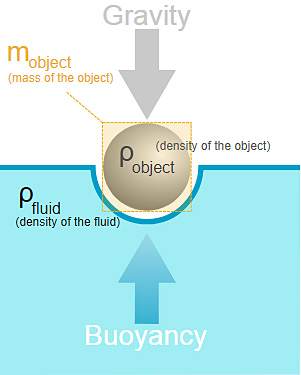The forces at work in buoyancy. The object floats at rest because the upward force of buoyancy is equal to the downward force of gravity.

## Archimedes’ principle

A metallic coin (an old British pound coin) floats in mercury due to the buoyancy force upon it and appears to float higher because of the surface tension of the mercury.

Archimedes’ principle is named after Archimedes of Syracuse, who first discovered this law in 212 B.C. For objects, floating and sunken, and in gases as well as liquids (i.e. a fluid), Archimedes’ principle may be stated thus in terms of forces:

Any object, wholly or partially immersed in a fluid, is buoyed up by a force equal to the weight of the fluid displaced by the object

— with the clarifications that for a sunken object the volume of displaced fluid is the volume of the object, and for a floating object on a liquid, the weight of the displaced liquid is the weight of the object.

More tersely: Buoyancy = weight of displaced fluid.

Archimedes’ principle does not consider the surface tension (capillarity) acting on the body, but this additional force modifies only the amount of fluid displaced and the spatial distribution of the displacement, so the principle that Buoyancy = weight of displaced fluid remains valid.

The weight of the displaced fluid is directly proportional to the volume of the displaced fluid (if the surrounding fluid is of uniform density). In simple terms, the principle states that the buoyancy force on an object is equal to the weight of the fluid displaced by the object, or the density of the fluid multiplied by the submerged volume times the gravitational acceleration, g. Thus, among completely submerged objects with equal masses, objects with greater volume have greater buoyancy. This is also known as upthrust.

Suppose a rock’s weight is measured as 10 newtons when suspended by a string in a vacuum with gravity acting upon it. Suppose that when the rock is lowered into water, it displaces water of weight 3 newtons. The force it then exerts on the string from which it hangs would be 10 newtons minus the 3 newtons of buoyancy force: 10 − 3 = 7 newtons. Buoyancy reduces the apparent weight of objects that have sunk completely to the sea floor. It is generally easier to lift an object up through the water than it is to pull it out of the water.

Assuming Archimedes’ principle to be reformulated as follows,then inserted into the quotient of weights, which has been expanded by the mutual volume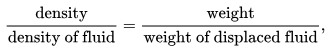yields the formula below. The density of the immersed object relative to the density of the fluid can easily be calculated without measuring any volumes.: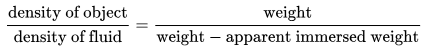(This formula is used for example in describing the measuring principle of a dasymeter and of hydrostatic weighing.)

Example: If you drop wood into water, buoyancy will keep it afloat.

Example: A helium balloon in a moving car. During a period of increasing speed, the air mass inside the car moves in the direction opposite to the car’s acceleration (i.e., towards the rear). The balloon is also pulled this way. However, because the balloon is buoyant relative to the air, it ends up being pushed «out of the way», and will actually drift in the same direction as the car’s acceleration (i.e., forward). If the car slows down, the same balloon will begin to drift backward. For the same reason, as the car goes round a curve, the balloon will drift towards the inside of the curve.

## Forces and equilibrium

The equation to calculate the pressure inside a fluid in equilibrium is: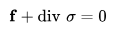where f is the force density exerted by some outer field on the fluid, and σ is the Cauchy stress tensor. In this case the stress tensor is proportional to the identity tensor: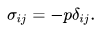Here δij is the Kronecker delta. Using this the above equation becomes: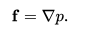Assuming the outer force field is conservative, that is it can be written as the negative gradient of some scalar valued function: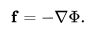Then: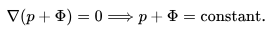Therefore, the shape of the open surface of a fluid equals the equipotential plane of the applied outer conservative force field. Let the z-axis point downward. In this case the field is gravity, so Φ = −ρfgz where g is the gravitational acceleration, ρf is the mass density of the fluid. Taking the pressure as zero at the surface, where z is zero, the constant will be zero, so the pressure inside the fluid, when it is subject to gravity, is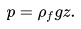So pressure increases with depth below the surface of a liquid, as z denotes the distance from the surface of the liquid into it. Any object with a non-zero vertical depth will have different pressures on its top and bottom, with the pressure on the bottom being greater. This difference in pressure causes the upward buoyancy force.

The buoyancy force exerted on a body can now be calculated easily, since the internal pressure of the fluid is known. The force exerted on the body can be calculated by integrating the stress tensor over the surface of the body which is in contact with the fluid: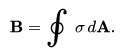The surface integral can be transformed into a volume integral with the help of the Gauss theorem: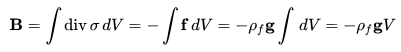where V is the measure of the volume in contact with the fluid, that is the volume of the submerged part of the body, since the fluid doesn’t exert force on the part of the body which is outside of it.

The magnitude of buoyancy force may be appreciated a bit more from the following argument. Consider any object of arbitrary shape and volume V surrounded by a liquid. The force the liquid exerts on an object within the liquid is equal to the weight of the liquid with a volume equal to that of the object. This force is applied in a direction opposite to gravitational force, that is of magnitude: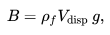where ρf is the density of the fluid, Vdisp is the volume of the displaced body of liquid, and g is the gravitational acceleration at the location in question.

If this volume of liquid is replaced by a solid body of exactly the same shape, the force the liquid exerts on it must be exactly the same as above. In other words, the «buoyancy force» on a submerged body is directed in the opposite direction to gravity and is equal in magnitude to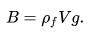The net force on the object must be zero if it is to be a situation of fluid statics such that Archimedes principle is applicable, and is thus the sum of the buoyancy force and the object’s weight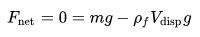If the buoyancy of an (unrestrained and unpowered) object exceeds its weight, it tends to rise. An object whose weight exceeds its buoyancy tends to sink. Calculation of the upwards force on a submerged object during its accelerating period cannot be done by the Archimedes principle alone; it is necessary to consider dynamics of an object involving buoyancy. Once it fully sinks to the floor of the fluid or rises to the surface and settles, Archimedes principle can be applied alone. For a floating object, only the submerged volume displaces water. For a sunken object, the entire volume displaces water, and there will be an additional force of reaction from the solid floor.

In order for Archimedes’ principle to be used alone, the object in question must be in equilibrium (the sum of the forces on the object must be zero), therefore;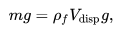and therefore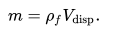showing that the depth to which a floating object will sink, and the volume of fluid it will displace, is independent of the gravitational field regardless of geographic location.

(Note: If the fluid in question is seawater, it will not have the same density (ρ) at every location. For this reason, a ship may display a Plimsoll line.)

It can be the case that forces other than just buoyancy and gravity come into play. This is the case if the object is restrained or if the object sinks to the solid floor. An object which tends to float requires a tension restraint force T in order to remain fully submerged. An object which tends to sink will eventually have a normal force of constraint N exerted upon it by the solid floor. The constraint force can be tension in a spring scale measuring its weight in the fluid, and is how apparent weight is defined.

If the object would otherwise float, the tension to restrain it fully submerged is: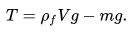When a sinking object settles on the solid floor, it experiences a normal force of: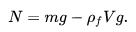Another possible formula for calculating buoyancy of an object is by finding the apparent weight of that particular object in the air (calculated in Newtons), and apparent weight of that object in the water (in Newtons). To find the force of buoyancy acting on the object when in air, using this particular information, this formula applies:

Buoyancy force = weight of object in empty space − weight of object immersed in fluid

The final result would be measured in Newtons.

Air’s density is very small compared to most solids and liquids. For this reason, the weight of an object in air is approximately the same as its true weight in a vacuum. The buoyancy of air is neglected for most objects during a measurement in air because the error is usually insignificant (typically less than 0.1% except for objects of very low average density such as a balloon or light foam).

### Simplified model

A simplified explanation for the integration of the pressure over the contact area may be stated as follows:

Consider a cube immersed in a fluid with the upper surface horizontal.

The sides are identical in area, and have the same depth distribution, therefore they also have the same pressure distribution, and consequently the same total force resulting from hydrostatic pressure, exerted perpendicular to the plane of the surface of each side.

There are two pairs of opposing sides, therefore the resultant horizontal forces balance in both orthogonal directions, and the resultant force is zero.

The upward force on the cube is the pressure on the bottom surface integrated over its area. The surface is at constant depth, so the pressure is constant. Therefore, the integral of the pressure over the area of the horizontal bottom surface of the cube is the hydrostatic pressure at that depth multiplied by the area of the bottom surface.

Similarly, the downward force on the cube is the pressure on the top surface integrated over its area. The surface is at constant depth, so the pressure is constant. Therefore, the integral of the pressure over the area of the horizontal top surface of the cube is the hydrostatic pressure at that depth multiplied by the area of the top surface.

As this is a cube, the top and bottom surfaces are identical in shape and area, and the pressure difference between the top and bottom of the cube is directly proportional to the depth difference, and the resultant force difference is exactly equal to the weight of the fluid that would occupy the volume of the cube in its absence.

This means that the resultant upward force on the cube is equal to the weight of the fluid that would fit into the volume of the cube, and the downward force on the cube is its weight, in the absence of external forces.

This analogy is valid for variations in the size of the cube.

If two cubes are placed alongside each other with a face of each in contact, the pressures and resultant forces on the sides or parts thereof in contact are balanced and may be disregarded, as the contact surfaces are equal in shape, size and pressure distribution, therefore the buoyancy of two cubes in contact is the sum of the buoyancies of each cube. This analogy can be extended to an arbitrary number of cubes.

An object of any shape can be approximated as a group of cubes in contact with each other, and as the size of the cube is decreased, the precision of the approximation increases. The limiting case for infinitely small cubes is the exact equivalence.

Angled surfaces do not nullify the analogy as the resultant force can be split into orthogonal components and each dealt with in the same way.

### Static stability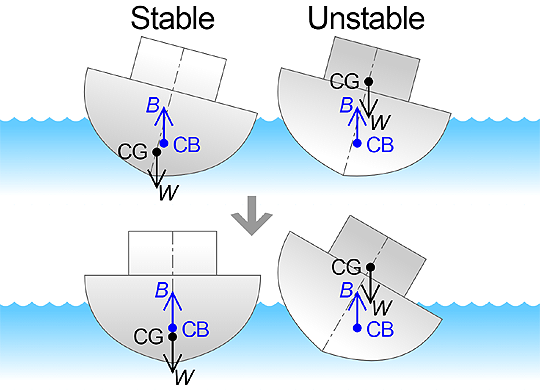A floating object is stable if it tends to restore itself to an equilibrium position after a small displacement. For example, floating objects will generally have vertical stability, as if the object is pushed down slightly, this will create a greater buoyancy force, which, unbalanced by the weight force, will push the object back up.

Rotational stability is of great importance to floating vessels. Given a small angular displacement, the vessel may return to its original position (stable), move away from its original position (unstable), or remain where it is (neutral).

Rotational stability depends on the relative lines of action of forces on an object. The upward buoyancy force on an object acts through the center of buoyancy, being the centroid of the displaced volume of fluid. The weight force on the object acts through its center of gravity. A buoyant object will be stable if the center of gravity is beneath the center of buoyancy because any angular displacement will then produce a ‘righting moment’.

The stability of a buoyant object at the surface is more complex, and it may remain stable even if the centre of gravity is above the centre of buoyancy, provided that when disturbed from the equilibrium position, the centre of buoyancy moves further to the same side that the centre of gravity moves, thus providing a positive righting moment. If this occurs, the floating object is said to have a positive metacentric height. This situation is typically valid for a range of heel angles, beyond which the centre of buoyancy does not move enough to provide a positive righting moment, and the object becomes unstable. It is possible to shift from positive to negative or vice versa more than once during a heeling disturbance, and many shapes are stable in more than one position.

## Compressible fluids and objects

The atmosphere’s density depends upon altitude. As an airship rises in the atmosphere, its buoyancy decreases as the density of the surrounding air decreases. In contrast, as a submarine expels water from its buoyancy tanks, it rises because its volume is constant (the volume of water it displaces if it is fully submerged) while its mass is decreased.

### Compressible objects

As a floating object rises or falls, the forces external to it change and, as all objects are compressible to some extent or another, so does the object’s volume. Buoyancy depends on volume and so an object’s buoyancy reduces if it is compressed and increases if it expands.

If an object at equilibrium has a compressibility less than that of the surrounding fluid, the object’s equilibrium is stable and it remains at rest. If, however, its compressibility is greater, its equilibrium is then unstable, and it rises and expands on the slightest upward perturbation, or falls and compresses on the slightest downward perturbation.

#### Submarines

Submarines rise and dive by filling large ballast tanks with seawater. To dive, the tanks are opened to allow air to exhaust out the top of the tanks, while the water flows in from the bottom. Once the weight has been balanced so the overall density of the submarine is equal to the water around it, it has neutral buoyancy and will remain at that depth. Most military submarines operate with a slightly negative buoyancy and maintain depth by using the «lift» of the stabilizers with forward motion.

#### Balloons

The height to which a balloon rises tends to be stable. As a balloon rises it tends to increase in volume with reducing atmospheric pressure, but the balloon itself does not expand as much as the air on which it rides. The average density of the balloon decreases less than that of the surrounding air. The weight of the displaced air is reduced. A rising balloon stops rising when it and the displaced air are equal in weight. Similarly, a sinking balloon tends to stop sinking.

#### Divers

Underwater divers are a common example of the problem of unstable buoyancy due to compressibility. The diver typically wears an exposure suit which relies on gas-filled spaces for insulation, and may also wear a buoyancy compensator, which is a variable volume buoyancy bag which is inflated to increase buoyancy and deflated to decrease buoyancy. The desired condition is usually neutral buoyancy when the diver is swimming in mid-water, and this condition is unstable, so the diver is constantly making fine adjustments by control of lung volume, and has to adjust the contents of the buoyancy compensator if the depth varies.

## DensityDensity column of liquids & solids: baby oil, alcohol (with red food coloring), vegetable oil, wax, water (with blue food coloring), & aluminum.

If the weight of an object is less than the weight of the displaced fluid when fully submerged, then the object has an average density that is less than the fluid and when fully submerged will experience a buoyancy force greater than its own weight. If the fluid has a surface, such as water in a lake or the sea, the object will float and settle at a level where it displaces the same weight of fluid as the weight of the object. If the object is immersed in the fluid, such as a submerged submarine or air in a balloon, it will tend to rise. If the object has exactly the same density as the fluid, then its buoyancy equals its weight. It will remain submerged in the fluid, but it will neither sink nor float, although a disturbance in either direction will cause it to drift away from its position. An object with a higher average density than the fluid will never experience more buoyancy than weight and it will sink. A ship will float even though it may be made of steel (which is much denser than water), because it encloses a volume of air (which is much less dense than water), and the resulting shape has an average density less than that of the water.

Text from Wikipedia is available under the Creative Commons Attribution/Share-Alike License; additional terms may apply.

Published in August 2018.

Please see some ads intermixed with other content from this site:Copyright 2004-2021 © by Airports-Worldwide.com, Vyshenskoho st. 36, Lviv 79010, Ukraine
Legal Disclaimer